# PSEB 3rd Class Maths Solutions Chapter 4 Division

Punjab State Board PSEB 3rd Class Maths Book Solutions Chapter 4 Division Textbook Exercise Questions and Answers.

## PSEB Solutions for Class 3 Maths Chapter 4 Division

PAGE 101:

Do you remember?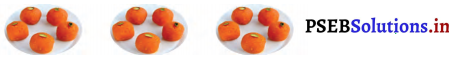(i) There are _______ ladoos in plates.
Solution.
15

(ii) Ladoos are distributed in _______ equal groups.
Solution.
3

(iii) There are _______ ladoos in each group.
Solution.
5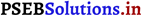PAGE 104:

Division by subtraction method: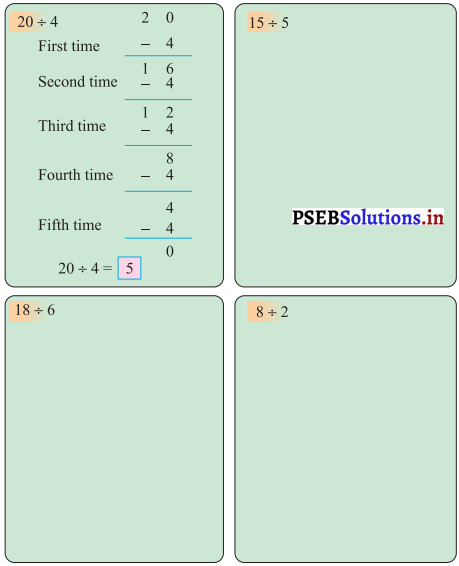Solution.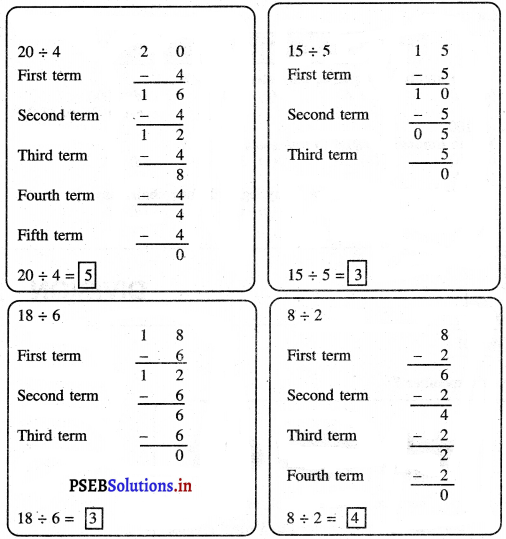.PAGE 105:
Let’s do: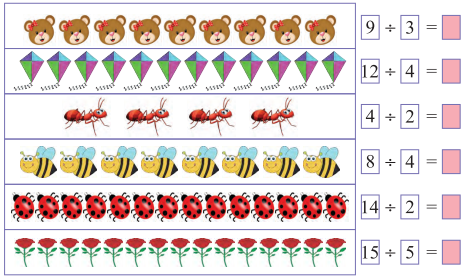Solution.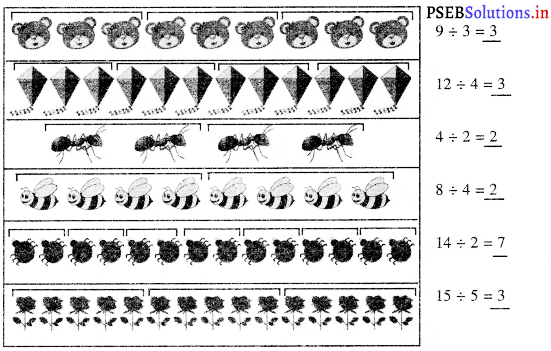PAGE 109:
Let’s do:

a. Divide 12 apples in 3 plates equally.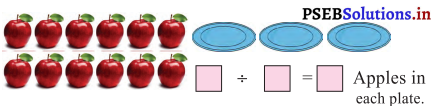Solution.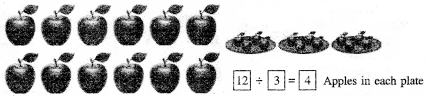b. Divide 8 ice creams among 2 children equally.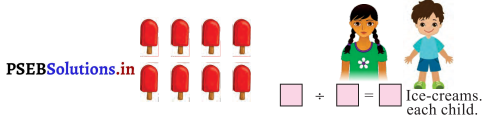Solution.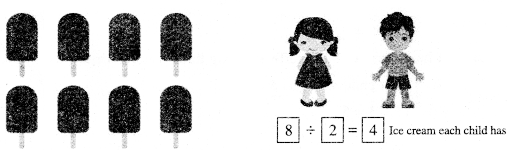PAGE 109:

Relation between multiplication and division: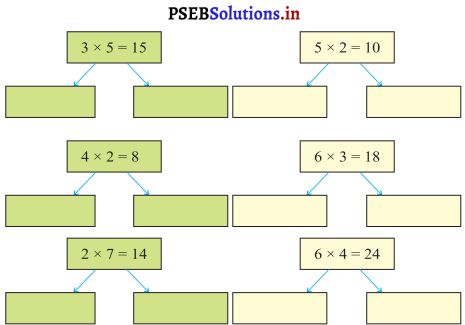Solution.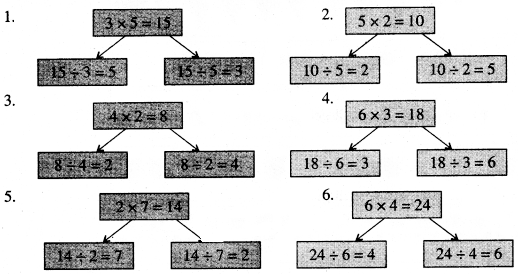PAGE 110:

Avneet has 15 toffees. He distributes these toffees equally among the five children.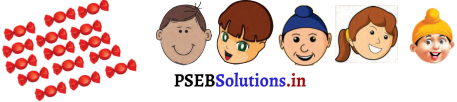(a) (i) Each child will get _______ toffees.
Solution.
3

(ii) 15 ÷ 5 = _______
Solution.
3

(b) If he distributes these toffees among three children then,
(i) Each child will get _______ toffees.
Solution.
5

(ii) 15 ÷ 3 = _______
Solution.
5Rasleen has 12 balloons. He distributes these balloons equally among 3 children.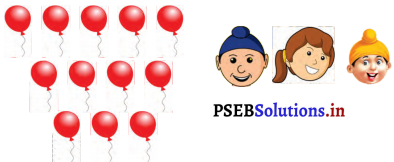(a) (i) Each child will get _______ balloons.
Solution.
4

(ii) 12 ÷ 3 = _______
Solution.
4

(b) If he distributes these balloons among 4 children then,
(i) Each child will get _______ balloons
Solution.
3

(ii) 12 ÷ 4 = _______
Solution.
3PAGE 111:
Division by using multiplication table:

Use multiplication table of 2 and divide: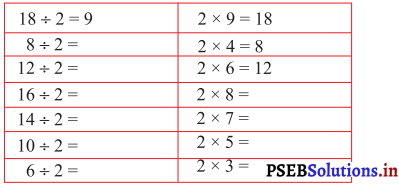Solution.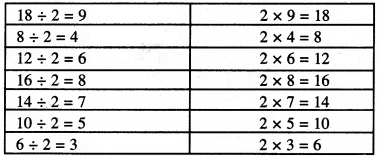Use multiplication table of 5 and divide :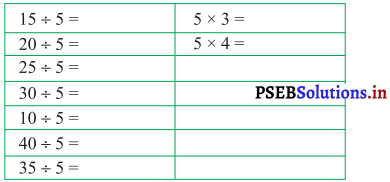Solution.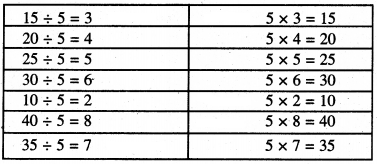Use multiplication table of 10 and divide: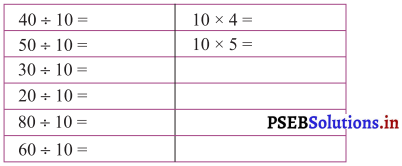Solution.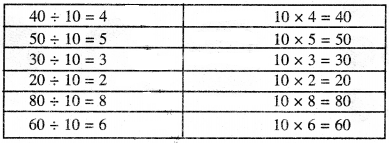PAGE 112:
Let’s do:

Question 1.
Fill in the blanks:
(i) 4 ÷ 4 = ___
Solution.
1

(ii) 3 ÷ ___ = 1
Solution.
3

(iii) 0 ÷ 5 = ___
Solution.
0

(iv) ___ ÷ 2 = 0
Solution.
0

(v) 2 ÷ 2 = ___
Solution.
1

(vi) 10 ÷ ___ = 1
Solution.
10PAGE 115:
Let’s do:

Divide 2-digits number by 1 digit number.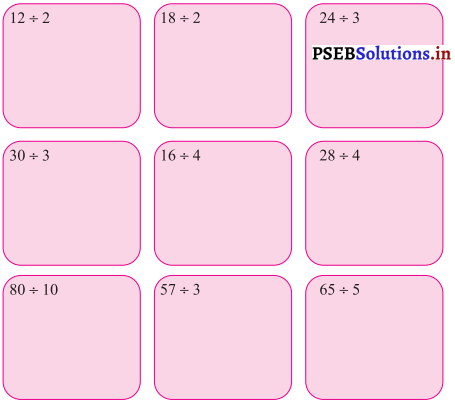Solution.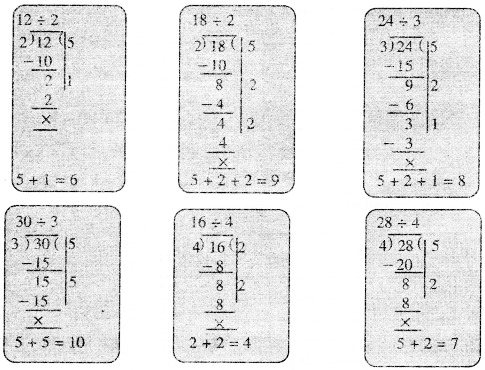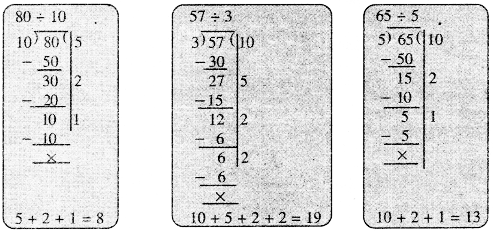Question 1.
Tania has 32 toffees. She wants to distribute these toffees equally between her two friends. How many toffees will each of her friend get ?
Solution.
Tania has toffees = 32
Number of friends = 2
Toffees each of her friend get = 32 ÷ 2 = 16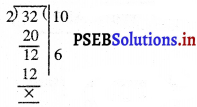10 + 6 = 16

Question 2.
Avneet has 10 erasers. He wants to distribute these erasers equally among his 5 friends. How many erasers will each friend get ?
Sol. Number of Erasers Avneet has = 10
No. of friends = 5
Number of Erasers each friend get = 2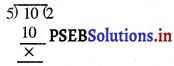Question 3.
There are 20 students in the class. They are sitting equally in 4 rows. How many students are sitting in each row ?
Solution.
Number of students in a class = 20
Number of rows =4
Number of students in each row = 5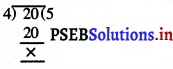PAGE 116:
Let’s Do:

Orally, double/half in following: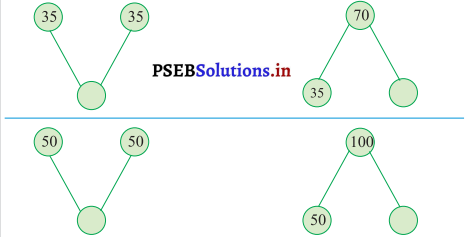Solution.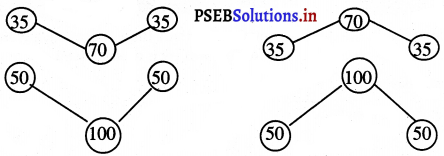PAGE 118:

Question 1.
Divide using repeated subtraction method :

(i) 15 ÷ 5
Solution.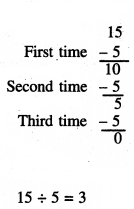(ii) 40 ÷ 10
Solution.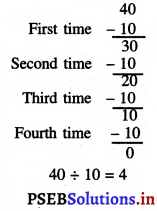(iii) 20 ÷ 4
Solution.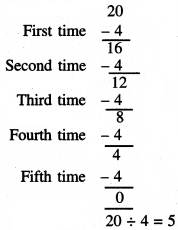Question 2.
Fill un the blanks: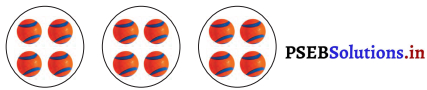(i) How many total balls are there? _______
Solution.
12

(ii) How many total groups are there? _______
Solution.
3

(iii) How many balls does each group has? _______
Solution.
4Question 3.
Write the division fact of the gien multiplication fact.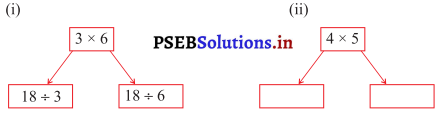Solution.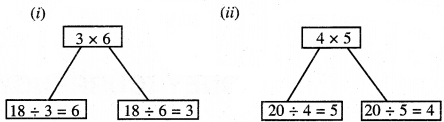Question 4.
Solve :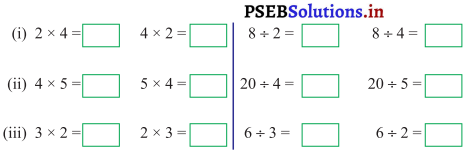Solution.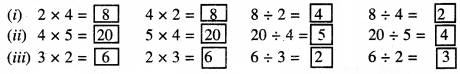Question 5.
Fill in the blanks :
(i) 15 ÷ 15 =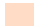Solution.
1

(ii) 18 ÷ 3 =Solution.
6

(iii) 0 ÷ 20 =Solution.
0

(iv) 32 ÷= 1
Solution.
32

(v)÷ 42 = 1
Solution.
42Question 6.
Divide:
(i) 48 ÷ 3 =
Solution.
16

(ii) 56 ÷ 4 =
Solution.
14

(iii) 70 ÷ 5 =
Solution.
14Multiple Choice Questions:

Question 1.
There are 15 Laddoos in a tray. We can pack 5 Laddoos in a box, then how many boxes are needed to pack all the laddoos in boxes ?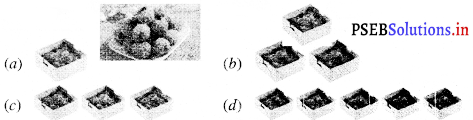Solution.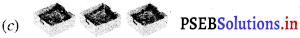Question 2.
Divide 12 apples in 3 plates equally. Apples in each plate are :
(a) 2
(b) 3
(c) 4
(d) 12
Solution.
(c) 4

Question 3.
Avneet has 15 toffees. He distributes these toffees equally among the five children. Each child will get……. toffees.
(a) 2
(b) 3
(c) 4
(d) 5
Solution.
(b) 3.Question 4.
Rasleen has 12 balloons. He distributes these balloons equally among 3 children. Each child will get:
(a) 2
(b) 3
(c) 4
(d) 1
Solution.
(c) 4.